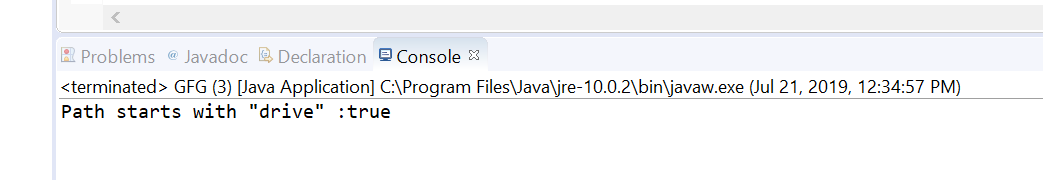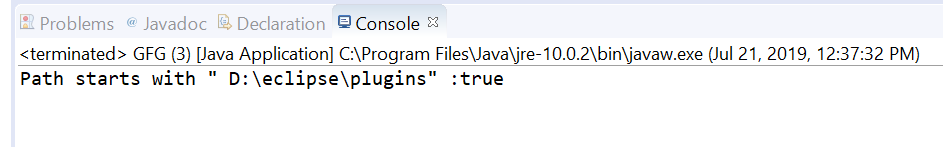Related Articles
Path startsWith() method in Java with Examples
• Last Updated : 23 Jul, 2019

startsWith() method of java.nio.file.Path usec to check if this path object starts with the given path or string which we passed as parameter.
There are two types of startsWith() methods.

1. startsWith(String other) method of java.nio.file.Path used to check if this path starts with a Path, constructed by converting the given path string which we passed as a parameter to this method. For example, this path “dir1/file1” starts with “dir1/file1” and “dir1”. It does not end with “d” or “dir”.

Syntax:

```default boolean startsWith(String other)
```

Parameters: This method accepts a single parameter other which is the given path string.

Return value: This method returns true if this path starts with the given path; otherwise false.

Exception: This method throws InvalidPathException If the path string cannot be converted to a Path.

Below programs illustrate startsWith(String other) method:
Program 1:

 `// Java program to demonstrate ` `// Path.startsWith(String other) method ` ` `  `import` `java.nio.file.Path; ` `import` `java.nio.file.Paths; ` `public` `class` `GFG { ` `    ``public` `static` `void` `main(String[] args) ` `    ``{ ` ` `  `        ``// create object of Path ` `        ``Path path ` `            ``= Paths.get(``"drive\\temp\\Spring"``); ` ` `  `        ``// create a string object ` `        ``String passedPath = ``"drive"``; ` ` `  `        ``// call startsWith() to check path object ` `        ``// starts with passedPath or not ` `        ``boolean` `check = path.startsWith(passedPath); ` ` `  `        ``// print result ` `        ``System.out.println(``"Path starts with \""` `                           ``+ passedPath + ``"\" :"` `                           ``+ check); ` `    ``} ` `} `

Output:2. startsWith(Path other) method of java.nio.file.Path used to check if this path starts with the given path as parameter to method or not.This method return true if this path starts with the given path; otherwise false.
This path starts with the given path if this path’s root component starts with the root component of the given path, and this path starts with the same name elements as the given path. If the given path has more name elements than this path then false is returned.
Whether or not the root component of this path starts with the root component of the given path is files system-specific. If this path does not have a root component and the given path has a root component then this path does not start with the given path.
If the given path is associated with a different FileSystem to this path then false is returned.

Syntax:

```boolean startsWith(Path other)
```

Parameters: This method accepts a single parameter other which is the given path.

Return value: This method return true if this path starts with the given path; otherwise false.

Below programs illustrate startsWith(Path other) method:
Program 1:

 `// Java program to demonstrate ` `// java.nio.file.Path.(Path other) method ` ` `  `import` `java.nio.file.Path; ` `import` `java.nio.file.Paths; ` `public` `class` `GFG { ` `    ``public` `static` `void` `main(String[] args) ` `    ``{ ` ` `  `        ``// create object of Path ` `        ``Path path ` `            ``= Paths.get(``"D:\\eclipse"` `                        ``+ ``"\\plugins"` `                        ``+ ``"\\javax.xml.rpc_1.1.0.v201209140446"` `                        ``+ ``"\\lib"``); ` ` `  `        ``// create a path object which we will pass ` `        ``// to startsWith method to check functionality ` `        ``// of startsWith(Path other) method ` `        ``Path passedPath ` `            ``= Paths.get( ` `                ``"D:\\eclipse"` `                ``+ ``"\\plugins"``); ` ` `  `        ``// call startsWith() to check path object ` `        ``// starts with passedPath or not ` `        ``boolean` `check = path.startsWith(passedPath); ` ` `  `        ``// print result ` `        ``System.out.println(``"Path starts with \" "` `                           ``+ passedPath + ``"\" :"` `                           ``+ check); ` `    ``} ` `} `

Output:References:

Attention reader! Don’t stop learning now. Get hold of all the important Java Foundation and Collections concepts with the Fundamentals of Java and Java Collections Course at a student-friendly price and become industry ready.

My Personal Notes arrow_drop_up
Recommended Articles
Page :